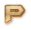# COA Representation Of Floating Number Online Test

Instructions:
• Total number of questions : 10.
• Time alloted : 10 minutes.
• Each question carry 1 mark.
• No Negative marks
• DO NOT refresh the page.
• All the best :-)

### What You Will Learn in

This COA Representation of Floating Number Test helps you to Boost your Knowledge in Computer Organization & Architecture. In this test, we will cover the topics in the form of questions like

• The decimal numbers represented in the computer are called as floating point numbers, as the decimal point floats through the number.
• Scale factors is defined as the numbers written to the power of 10 in the representation of decimal numbers.
• Mantissa is called as the sign followed by the string of digits.
• Single-precision is known as the 32 bit representation of the decimal number.